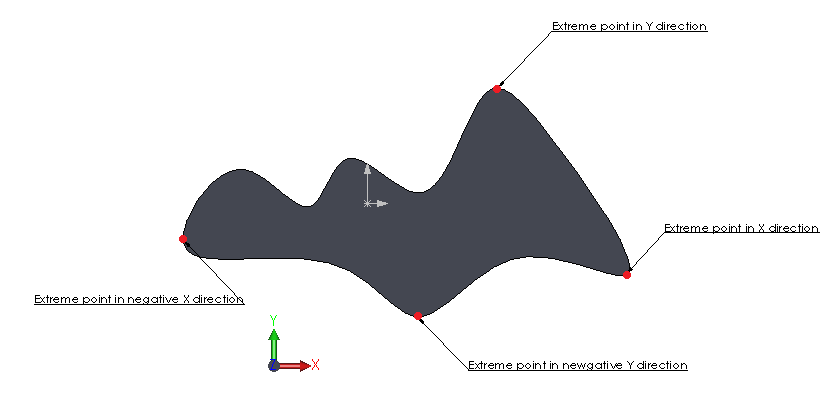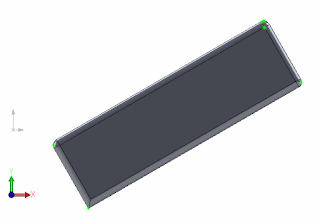# Macro gets extreme points on body using SOLIDWORKS API

Extreme points are usually used to find the most remote points in the specified directions. The points can be found using the IBody2::GetExtremePoint SOLIDWORKS API.

This function expects direction vector as an input and returns the X, Y, Z coordinate of the extreme point in this direction as out parameters.

It is not required to specify the point on the vector when defining the direction. This function is usually used to find the bounding dimension of the body, especially when the body orientation is not aligned with global XYZ coordinate as it is not required to reorient the body to find its best fit bounding box.

Unlike bounding box returned via IBody2::GetBodyBox or any other bounding box function extreme points are precise which means that this data can be used for comparison and calculation purposes.

Image below demonstrates typical extreme points in multiple directions of the model.Extreme points of the body in +X,-X,+Y and -Y directions

The following code example will find the extreme points of the selected body in XYZ directions and create a sketch points.Sketch points created in the extreme directions of the body

```Dim swApp As SldWorks.SldWorks
Dim swModel As SldWorks.ModelDoc2
Dim swSelMgr As SldWorks.SelectionMgr

Sub main()

Set swApp = Application.SldWorks

Set swModel = swApp.ActiveDoc

If Not swModel Is Nothing Then

Set swSelMgr = swModel.SelectionManager

If swSelMgr.GetSelectedObjectType3(1, -1) = swSelectType_e.swSelSOLIDBODIES Then

Dim swBody As SldWorks.Body2

Set swBody = swSelMgr.GetSelectedObject6(1, -1)

swModel.ClearSelection2 True

swModel.SketchManager.Insert3DSketch True
swModel.SketchManager.AddToDB = True

Dim vDirs(5) As Variant
vDirs(0) = Array(1, 0, 0)
vDirs(1) = Array(0, 1, 0)
vDirs(2) = Array(0, 0, 1)
vDirs(3) = Array(-1, 0, 0)
vDirs(4) = Array(0, -1, 0)
vDirs(5) = Array(0, 0, -1)

Dim i As Integer

For i = 0 To UBound(vDirs)

Dim x As Double
Dim y As Double
Dim z As Double

swBody.GetExtremePoint vDirs(i)(0), vDirs(i)(1), vDirs(i)(2), x, y, z
swModel.SketchManager.CreatePoint x, y, z

Next

swModel.SketchManager.AddToDB = False
swModel.SketchManager.Insert3DSketch True

Else

MsgBox "Please select solid body"

End If

Else

MsgBox "Please open part or assembly"

End If

End Sub

```